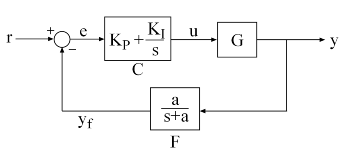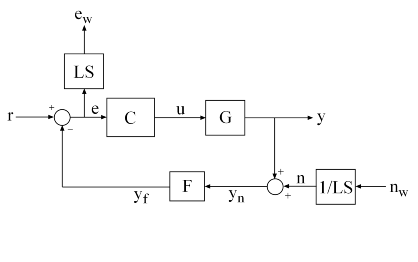# hinfstruct

H tuning of fixed-structure controllers

## Syntax

``CL = hinfstruct(CL0)``
``````[CL,gamma,info] = hinfstruct(CL0)``````
``````[CL,gamma,info] = hinfstruct(CL0,options)``````
``````[C,gamma,info] = hinfstruct(P,C0,options)``````

## Description

The `hinfstruct` command extends classical H synthesis (see `hinfsyn`) to fixed-structure control systems. If you are unfamiliar with constructing weighting functions to capture design requirements for H synthesis, use `systune` or `looptune` instead.

example

````CL = hinfstruct(CL0)` tunes the free parameters of the tunable `genss` model `CL0`. This tuning minimizes the H∞ norm of the closed-loop transfer function modeled by `CL0`. The model `CL0` represents a closed-loop control system that includes tunable components such as controllers or filters. `CL0` can also include weighting functions that capture design requirements.```

example

``````[CL,gamma,info] = hinfstruct(CL0)``` returns `gamma` (the minimum H∞ norm) and a data structure `info` with additional information about each optimization run.```

example

``````[CL,gamma,info] = hinfstruct(CL0,options)``` specifies additional options for the optimizer using `hinfstructOptions`.```
``````[C,gamma,info] = hinfstruct(P,C0,options)``` tunes the parametric controller blocks `C0`. This tuning minimizes the H∞ norm of the closed-loop system `CL0 = lft(P,C0)`. To use this syntax, express your control system and design requirements as a Standard Form model, as in the following illustration.`P` is a numeric LTI model that includes the fixed elements of the control architecture. `P` can also include weighting functions that capture design requirements. `C0` can be a single tunable component (for example, a Control Design Block or a `genss` model) or a cell array of multiple tunable components. `C` is a parametric model or cell array of parametric models of the same type or types as `C0`.```

## Examples

collapse all

Tune the controller elements of the following control system.The control elements are `C`, which is a `PI` controller with two free parameters, and `F`, which is a low-pass filter in the feedback path with one free parameter. For this example, load the plant `G`, a ninth-order model of the head disk assembly (HDA) in a hard disk drive.

```load hinfstruct_demo G bode(G), grid```Tune the free parameters of this control system so that the head position `y` tracks a step change `r` with a response time of about 1 ms, little or no overshoot, and no steady-state error.

First, create the tunable elements. Use a `tunablePID` object to parameterize the PI block, and specify the filter `F0` as a transfer function depending on a tunable real parameter `a`.

```C0 = tunablePID('C','pi'); a = realp('a',1); F0 = tf(a,[1 a]);```

`F0` is a `genss` model.

Next, express the design goals as weights on the plant model, and append them to the closed-loop system. For reasons described in detail in Fixed-Structure H-infinity Synthesis with hinfstruct, the following configuration of weighting functions achieves the design requirements for this problem.Here, `LS` is the desired shape of the open-loop response $L\left(s\right)=F\left(s\right)G\left(s\right)C\left(s\right)$.

```wc = 1000; % target crossover s = tf('s'); LS = (1+0.001*s/wc)/(0.001+s/wc);```

As discussed in Fixed-Structure H-infinity Synthesis with hinfstruct, the design requirements are satisfied if the ${Η}_{\infty }$ norm of this control structure is less than 1.

Use `connect` to construct a `genss` model representing this control structure.

```% Label the block I/Os Wn = 1/LS; Wn.u = 'nw'; Wn.y = 'n'; We = LS; We.u = 'e'; We.y = 'ew'; C0.u = 'e'; C0.y = 'u'; F0.u = 'yn'; F0.y = 'yf'; % Specify summing junctions Sum1 = sumblk('e = r - yf'); Sum2 = sumblk('yn = y + n'); % Connect the blocks together T0 = connect(G,Wn,We,C0,F0,Sum1,Sum2,{'r','nw'},{'y','ew'});```

You can now use `hinfstruct` to find tuned values of the tunable parameters in `C0` and `F0` that minimize the ${Η}_{\infty }$ norm of `T0`. To reduce the risk of finding local minima, run six optimizations, started from randomized initial values for `C0` and `F0`. The `RandomStart` option of `hinfstructOptions` specifies how many additional optimizations to run beyond the default one.

```rng('default') opt = hinfstructOptions('Display','final','RandomStart',5); T = hinfstruct(T0,opt);```
```Final: Peak gain = 3.88, Iterations = 67 Final: Peak gain = 597, Iterations = 191 Some closed-loop poles are marginally stable (decay rate near 1e-07) Final: Peak gain = 596, Iterations = 179 Some closed-loop poles are marginally stable (decay rate near 1e-07) Final: Peak gain = 1.91, Iterations = 91 Final: Peak gain = 1.56, Iterations = 99 Final: Peak gain = 1.56, Iterations = 94 ```

The best closed-loop gain is about 1.56, so the constraint $‖T{‖}_{\infty }<1$ is nearly satisfied. The `hinfstruct` command returns the tuned closed-loop transfer $T\left(s\right)$. To validate the design, plot the tuned open-loop response `L = F*G*C` and compare it with the target loop shape `LS`. To compute `L`, use `getBlockValue` to get the tuned value of $C\left(s\right)$ and use `getValue` to evaluate the filter $F\left(s\right)$ for the tuned value of $a$.

```C = getBlockValue(T,'C'); F = getValue(F0,T.Blocks); % Propagate tuned parameters from T to F L = G*C*F; bode(LS,'r--',G*C*F,'b',{1e1,1e6}), grid, title('Open-Loop Response'), legend('Target','Actual')```The 0 dB crossover frequency and overall loop shape are as expected. For further analysis of the result, see Fixed-Structure H-infinity Synthesis with hinfstruct.

## Input Arguments

collapse all

Closed-loop system with tunable elements, specified as a `genss` model. This model describes the closed-loop transfer function of a control system, incorporating appropriate weighting functions on the plant inputs and outputs to capture your design requirements. For more information about selecting weights for H tuning, see Formulating Design Requirements as H-Infinity Constraints.

`CL0` includes both the fixed and tunable components of the control system. You represent the tunable components of the control system using tunable control design blocks, which are stored in the `CL0.Blocks` property of the `genss` model. For more information about constructing this generalized model, see Build Tunable Closed-Loop Model for Tuning with hinfstruct. `hinfstruct` tunes the tunable elements of `CL0` to minimize the H norm.

Fixed elements of the control architecture, specified as a numeric LTI model such as a state-space (`ss`) model. `P` is the plant (along with any weighting functions to capture design requirements) that results from expressing your system in standard form, pulling any tunable elements into the block-diagonal controller as shown in the following diagram.You can obtain `P` in two ways:

• In MATLAB®, model the fixed elements of your control system as numeric LTI models. Then, use block-diagram building functions (such as `connect` and `feedback`) to build `P` from the modeled components. Also, include any weighting functions that represent your design requirements.

• If you have a Simulink® model of your control system and have Simulink Control Design™, use `linlft` (Simulink Control Design) to obtain a linear model of the fixed elements of your control system. The `linlft` command linearizes your Simulink model, excluding specified Simulink blocks (the blocks that represent the controller elements you want to tune). If you use weighting functions to represent your design requirements, connect them in series with the linear model of your plant to obtain `P`.

`P` can be a continuous-time or discrete-time model. In discrete time, the sample time must be specified (`Ts` ≠ –1), and must match the sample time of `C0`.

Tunable elements of the control architecture in standard form, specified as one of the following:

• A tunable control design block such as `tunableSS`, `tunableGain`, or `tunablePID`

• A generalized state-space (`genss`)

• A cell array in which each entry is a tunable block or `genss` model

For more information and examples of creating tunable models, see Models with Tunable Coefficients.

`C0` can be a continuous-time or discrete-time model, as long as the sample time matches that of `P`.

Algorithm options, specified as an `hinfstructOptions` options set. For information about available options, see `hinfstructOptions`.

## Output Arguments

collapse all

Tuned closed-loop system, returned as a generalized state-space (`genss`) model. `CL` is the tuned version of `CL0`.

The `hinfstruct` command tunes the free parameters of `CL0` to achieve a minimum H norm. `CL.Blocks` contains the same control design blocks as `CL0.Blocks`, except that in `CL`, the parameters have tuned values.

To access the tuned parameter values, use `getValue`. You can also access them directly in `CL.Blocks`.

Tuned controller elements, returned as a tunable control design block, a `genss` model, or a cell array of tunable blocks or tunable models. `C` is returned in the same format as `C0`, and contains the same tunable blocks, except that in `C`, the parameters have tuned values.

Best achieved H norm of the closed-loop system, returned as a positive scalar.

When you set the `hinfstructOptions` option `RandomStarts` to a nonzero value, `hinfstruct` performs more than one minimization run. In that case, `gamma` is the smallest H norm achieved over all runs.

Detailed results from each optimization run, returned as a structure, or a structure array if the `hinfstructOptions` option `RandomStarts` is nonzero. In that case, each entry in the array is the results structure for the corresponding optimization run. The fields of `info` are:

• `Objective` — Minimum H norm value for each run. When `RandomStarts` = 0, `Objective` = `gamma`.

• `Iterations` — Number of iterations before convergence for each run.

• `TunedBlocks` — Tuned control design blocks for each run. `TunedBlocks` differs from `C` in that `C` contains only the result from the best run. When `RandomStarts` = 0, `TunedBlocks` = `C`.

## Tips

• `hinfstruct` is related to `hinfsyn`, which also uses H techniques to design a controller for a MIMO plant. However, unlike `hinfstruct`, `hinfsyn` imposes no restriction on the structure and order of the controller. For that reason, `hinfsyn` always returns a smaller `gamma` than `hinfstruct`. You can therefore use `hinfsyn` to obtain a lower bound on the best achievable performance.

• Using `hinfstruct` requires some familiarity with H techniques. You must express your design requirements as frequency-weighting functions on plant inputs and outputs, as described in Formulating Design Requirements as H-Infinity Constraints. For a simpler approach to fixed-structure tuning, use `systune` or `looptune`.

## Algorithms

`hinfstruct` uses specialized nonsmooth optimization techniques to enforce closed-loop stability and minimize the H norm as a function of the tunable parameters. These techniques are based on the work in .

`hinfstruct` computes the H norm using the algorithm of  and structure-preserving eigensolvers from the SLICOT library. For more information about the SLICOT library, see http://slicot.org.

 Apkarian, Pierre, and Dominikus Noll. "Nonsmooth H Synthesis." IEEE Transactions on Automatic Control, 51, no. 1 (January 2006): 71–86. https://doi.org/10.1109/TAC.2005.860290.

 Bruinsma, N.A., and M. Steinbuch. "A Fast Algorithm to Compute the H Norm of a Transfer Function Matrix." Systems & Control Letters, 14, no.4 (April 1990): 287–93. https://doi.org/10.1016/0167-6911(90)90049-Z.